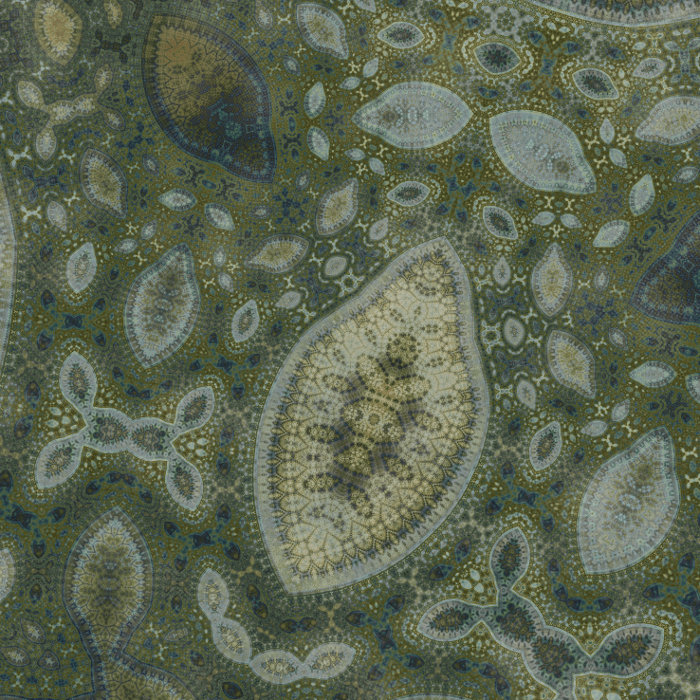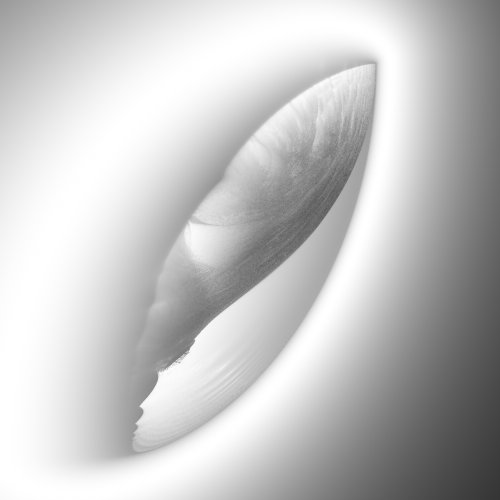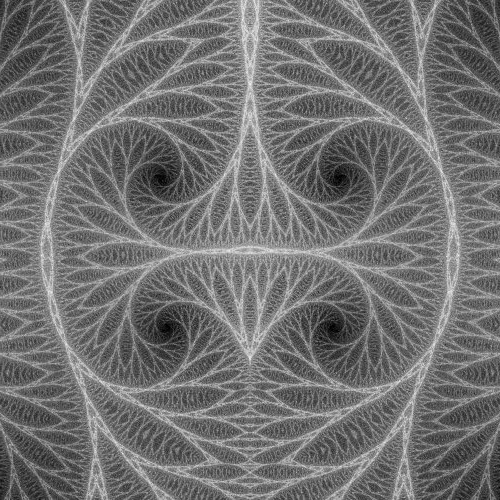### 20110129-1

2011, digital image and unique archival pigment print

The zoomable versions of the works are currently unavailable.

Back to the index

February 27th 2011

## Ducks and butterflies

Recently I've been using a different type of algorithm. It is still related to pattern piling, but I'll explain this in another post. Here I just want speak a bit about the algorithm.

A few years ago, a new type of fractal imagery appeared (or at least I hadn't encountered it before), involving fractal patterns apparently filling densely the plane. See this picture or this one for typical examples. Some beautiful images using the same type of patterns can be found in misterxz gallery at deviantart.

Dense fractal patterns are exactly what I am after, so I was naturally very interested in these new techniques. I don't know who discovered them, but for some time it was popular among users of Fractal Explorer, with a formula called "Thalis". Then Ed Algra wrote a few formulas called "Ducky" for Ultra Fractal that reproduced the same type of patterns. In an attempt to simply the algorithms as much as possible in order to understand how they are working, I ended up with the following algorithm that reproduces most of the patterns. Because I like Ducky and lack imagination for names, I called it Ducks. Compared to some other Ducky-like algorithms has the nice property that the patterns are never stretched, and that no branch cut ever appears.

Let z be a complex number and define Iabs(z) to be equal to z if the imaginary part of z is positive and its complex conjugate if it is negative. Geometrically, this transformation replace the lower half complex plane by the mirror image of the upper half complex plane. Now for each complex number p in the complex plane, iterate the following function for a fixed number of iterations (typically 50 to 100):

z = log(Iabs(z)+p)

Here the branch cut of the logarithm has been chosen to lie on the negative real axis. Color the pixel corresponding to p according to the mean of the magnitude of z, summed over all iterations. This type of algorithm, where a function is iterated, is called a dynamical system in mathematics and is a well known source of fractals. If you implement this algorithm, you will get something like this:The Ducks Mandelbrot-type fractal. The top right corner of the structure lies at 1, while the bottom left lies at -1 - pi*i.

It might not be obvious in the picture above, but there are some very intricate structures between the two curved arcs. Note that this fractal involves iterating a function that depends on the original pixel coordinates p. This is a "Mandelbrot type fractal". Indeed, to draw the Mandelbrot set, we have to iterated z = z^2 + p. For each point c in the complex plane, there is a Julia set, that we can draw by iterating the same function, but replacing p by the number c, that is fixed for all pixels. Remarkably, the Julia set based on c displays structures similar to the ones that can be seen in the Mandelbrot set around c. Similarly, we can draw Julia Ducks fractals by replacing p by a fixed number c in the algorithm above. Now if we choose c to lie in the middle region where the intricate structures lie, it turns out that we get infinitely extended fractal patterns that fill the plane densely. Here are five choices of c, bundled up in an animated gif. For aesthetic reason, I turned the pattern by 90° so that the real axis is vertical.Five examples of Ducks Julia-type fractals, chosen among those that fills the plane densely with fractal patterns.

In the standard Mandelbrot fractals, the more you zoom, the more iterations you have to perform to keep the same level of details. The same is true about the Ducks Julia fractal... except if you're zooming about zero! You can zoom as deep as you want and keep the same level of detail with the same number of iteration. It also works if you zoom out. Here is a zoom on a Ducks Julia about the origin, using Damien Jones' whoosh flash applet.

In some sense, it means the Ducks Julia fractal lives naturally on an infinite cylinder, rather than on the plane. (To visualize this cylinder, look at the animation above and imagine you're actually travelling inside an infinitely long cylinder.)

Now let us try to understand in a very heuristic way how the algorithm creates what we are seeing... The "mirror" Iabs(.) is an essential ingredient, that mainly has two functions. First, it removes the branch cut in the logarithm. Indeed, while the logarithm is a discontinuous function, Iabs(log(.)) is a continuous one. So at least for a finite number of iterations, the final pattern is continuous (even if it may tend to a discontinuous one as the number of iterations increases). Second, it is a two to one function: both z and its complex conjugate have the same image. This means that the orbits of the dynamical system merge two by two at each iteration, and this fact is responsible for the numerous "local mirror symmetries" that are visible in the pattern. I would suspect it is also responsible for the high degree of self-similarity of these patterns. Another crucial ingredient is the logarithm. It tends to bring back all the orbits around complex numbers of magnitude close to 1, ensuring that they will be noticeably affected by the addition of c. Supposedly, this is one of the reasons why it is displaying structures everywhere... but this doesn't explain why these structures are present only in a limited range of values for the Julia parameter. All of this is of course very vague, I wish I understood better what's going on. But very roughly put, after looking at other algorithms giving the same type of fractal patterns, it seems they arise when a "mirror" map like Iabs(.) is combined with some random conformal transformations. (If the transformations are not conformal, the pattern will tend to stretch unaesthetically...)

What about butterflies?, you may ask. Very interestingly, Jonathan McCabe came up with similar patterns in 2006, along a different route. I read about this on the Generator.x blog. His idea was the following. Take a square, and choose a number of random lines cutting the square and order them. For each point of the square, compute its images under the sequence of mirror symmetries about the sequence of random lines. Then color the result by performing an average over all the image points. He called this algorithm "Butterfly Origami". In essence, this is very similar to the Duck-like algorithms, except that instead of choosing random mirror axis, we are fixing the axis and performing some conformal transformation on the point. He also made some very nice videos by slowly moving the random axes. Check them here. (Look for "Butterfly Origami".) I've included one of them below.

I made a collection containing the works using Ducks. In a future post, I'll explain what this has to do with pattern piling...

[Updates: A quick study of a few orbits of the Ducks dynamical system showed something that could have been guessed... The "smooth" regions in the Mandelbrot version of the Ducks fractal pictured above correspond to orbits that quickly converge to a fixed point. The interesting jagged regions correspond to orbits that seem more chaotic, although many of them seem to settle down near attractors of various shapes... Maybe there will be a blog post in the future about this.

Also, check Ed Algra's comment below for more on the history of this algorithm.

Finally, check this blog post for very deep zooms on Ducks fractals.]